Do you like the article?
Share it with others -

Use new possibilities of MetaTrader 5

8 June 2017, 10:04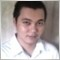28
15 601

### Introduction

In the previous article, Cross-Platform Expert Advisor: Order Manager, we have shown the COrderManager class, and how it can be used to automate the process of opening and closing trades. In this article, we will use roughly the same principles in order to automate the processes for the generation of signals. This can be achieved through the CSignal class, and its container, the CSignals class. This article details an implementation of these class objects.

### Objectives

This CSignal and CSignal classes mentioned in this article have the following objectives:

• The implementation should be compatible with MQL4 and MQL5.
• Automate most of the processes associated with the evaluation of trade signals
• The implementation should be simple

Both CSignal and its container, CSignals, are responsible for evaluating the overall signal given the current state of the market. The trading signals are divided into two main groups: entry and exit signals. In order for an entry signal to result to the EA executing a trade, that signal and all the other entry signals should agree to the same direction (all long or all short). On the other hand, for exit signals, each signal is independent on its own, and can influence the final outcome based on its output alone. The exit signals are also evaluated cumulatively, so for example, if signal 1 gives a close all sell condition and signal 2 gives a close all buy condition, the final outcome would be to close all trades.

### Signal Types

The EA has four different signal types, which are interpreted by the signal objects (and consequently, the EA) based on how it is used (for entry or exit). The following table shows the signal types, their values, and how they are interpreted depending on their target usage:

Signal Type
Value EntryExit
CMD_VOID-1
Invalidates all other signals
CMD_NEUTRAL0
Ignored
Ignored
CMD_LONG1
Go long
CMD_SHORT2
Go short

The signal types CMD_LONG and CMD_SHORT are pretty self-explanatory, so we will focus more on the other two signal types.

CMD_VOID has an integer value of -1, and refers to a signal that is in strong disagreement. For entry signals, a signal giving this output invalidates the output of all the other entry signals. This means that the output of this signal is mandatory, and a no-trade condition given by this signal will result to a no-trade condition for all the other signals, regardless of their actual outputs and whether or not all the other signals agree to the same direction. As an example, consider the following case of three signals for entry:

```Signal 1: CMD_VOID

Signal 2: CMD_LONG

Signal 3: CMD_SHORT

Final: CMD_VOID```

In this case, we can see that signal 1 will eventually invalidate the other two signals, with the final outcome to be CMD_VOID. But also notice that signals 2 and 3 do not agree to the same direction, and thus, whatever the value of signal 1 in this case will still result in a no-trade situation for the EA.

Let us now consider a slightly modified case as shown in the following:

```Signal 1: CMD_VOID

Signal 2: CMD_LONG

Signal 3: CMD_LONG

Final: CMD_VOID```

In this instance, signals 2 and 3 agree to the same direction (long), but signal 1 is void. Thus, the overall signal is a no-trade situation. Signal 1 is given more weight when it gave a void signal, even if signals 1 and 2 are in agreement.

When looking for an exit signal, on the other hand, the second example results to the exit of all positions: all the three signals agree to closing long positions, while signal 1 sends a message to close both long and short positions. Since all the exit signals are evaluated cumulatively, the final outcome would be to close all trades.

CMD_NEUTRAL has an integer value of 0, and is used to denote a refusal to give a signal. This is roughly equivalent to "abstinence" in an election process. A signal giving a neutral output surrenders its privilege to influence the final outcome of the signal, and leaves the decision to the rest of the signals. If, however, there is only one signal and that signal gave a neutral stance, then it would result to a no-entry and no-exit situation in the EA, which would also the same case as multiple signals not agreeing to the same direction.

Let us now make an example using CMD_NEUTRAL by slightly modifying the first example in this section:

```Signal 1: CMD_NEUTRAL

Signal 2: CMD_LONG

Signal 3: CMD_SHORT

FINAL: CMD_VOID```

In our third example, signal 1 gives a neutral position. In this case, only signals 2 and 3 will be considered for the final signal output. And since signals 2 and 3 do not agree to the same direction, the final outcome would be a no-trade situation (CMD_NEUTRAL).

The case is different when the remaining signals agree to the same direction. In our fourth example (shown below), the first signal is neutral and the remaining signals agree to the same direction. In this case, signal 1 is ignored, and signals 2 and 3 are evaluated, resulting to giving a buy signal as the final outcome.

```Signal 1: CMD_NEUTRAL

Signal 2: CMD_LONG

Signal 3: CMD_LONG

FINAL: CMD_LONG```

Note that the order of the signals do not matter, and the following set of signals:

```Signal 1: CMD_NEUTRAL

Signal 2: CMD_LONG

Signal 3: CMD_LONG

Signal 4: CMD_NEUTRAL

Signal 5: CMD_LONG

Signal 6: CMD_NEUTRAL```

will still result to an overall signal of CMD_LONG, not CMD_NEUTRAL.

When used as an exit signal, a signal having an output of CMD_NEUTRAL will not influence the final outcome of the final exit signal. At that particular moment, it would be as if that exit signal did not exist at all as far as determining the final signal is concerned.

It is also worth noting here that the assigned value of CMD_NEUTRAL is 0, and that we are using this custom enum as a substitute for ENUM_ORDER_TYPE. Using this custom enumeration has certain advantages. First, we can better customize how the signal is interpreted. Another advantage is that we can prevent the accidental execution of trades from uninitialized variables. For example, ORDER_TYPE_BUY has an integer value of 0. If we have an EA that directly passes an int variable directly to a method that processes trade requests, and that variable is not initialized or not reassigned to another value (most probably unintentional), then the default value is 0, which would then result to the entry of a buy order. On the other hand, with the custom enumeration, such an accident will never happen, as a value of zero for the variable will always result to a no-trade situation.

### Comparison with CExpertSignal

CExpertSignal evaluates the overall direction as follows:

1. Calculate its own direction and save to a variable named m_direction
2. For each of its filters
1. Gets its direction
2.  Adds the direction to m_direction (subtract, if the particular filter is inverted)
3. If the final value of m_direction exceeds the threshold, give a trade signal

Using this method, we can infer that the more positive the value of m_direction is, the more the signals evaluate that the price is likely to rise (increases the chance of exceeding the threshold value). Likewise, the more negative the value of m_direction is, the more the signals tend to predict that the price will fall. The threshold value is always positive, and so the absolute value of m_direction is used when checking for a signal for short.

The signal objects presented in this article can be considered a simplified version of CExpertSignal. However, rather than evaluating the signal and its filters collectively using arithmetic operations, each signal is evaluated separately. This is a less versatile approach, but gives the trader or programmer greater control on the extent by which each individual signal can influence the final outcome of the signal.

### Phases

#### OnInit and OnDeinit

The initialization phase of each signal would often deal with the creation and initialization of the indicators it would use, as well as the additional class members (if there are any) that maybe needed by the various methods found in the class object. At the de-initialization, the indicators instances will need to be deleted.

#### OnTick

1. Preliminary Phase (Calculation) - under this phase, the values needed for the calculation (signal checking) are updated.
2. Main Phase, or Signal Checking - during the main phase, the actual output of the signal is determined. Preferably, the body of this method should only have a single line of code, to improve code readability (see at a glance what the signal actually does).
3. Final or Update Phase - in some signals, there can exist certain members that can only be updated only after the actual signal checking has been performed. An example of this would be the tracking of the value of the previous bid price, possibly for comparing with the current bid price or some other value (possibly from a chart object or indicator output). Updating the variable that stores the previous bid price during the preliminary phase would make no sense since that would make its value always equal to the current bid price upon signal checking.

It is worth noting that MQL5 has a ticks array, while MQL4 does not have this feature. Nevertheless, MQL4 is the limiting factor here, and so the code should be made to adhere to MQL4 standards to ensure cross-platform compatibility, if splitting the implementation is the more rigorous option (which is true in this case).

### Implementation

#### CSignal Class

Prior to checking for any trade signal, it is customary to first refresh the data needed for the calculation. This is accomplished by the Refresh method of the CSignal class, where indicators (also, time-series data) are refreshed to their latest values. The following code snippet shows the Refresh method of CSignal:

```bool CSignalBase::Refresh(void)
{
for(int i=0;i<m_indicators.Total();i++)
{
CSeries *indicator=m_indicators.At(i);
if(indicator!=NULL)
indicator.Refresh(OBJ_ALL_PERIODS);
}
return true;
}```

The actual call to the Refresh method of CSignal is made within the Check method of the same class. As shown in the code below, the method would cease further processing if it was not able to refresh the data (which can lead to inaccurate signals).

```void CSignalBase::Check(void)
{
if(!Active())
return;
if(!Refresh())
return;
if(!Calculate())
return;
int res=CMD_NEUTRAL;
if(LongCondition())
{
if (Entry())
m_signal_open=CMD_LONG;
if (Exit())
m_signal_close=CMD_LONG;
}
else if(ShortCondition())
{
if (Entry())
m_signal_open=CMD_SHORT;
if (Exit())
m_signal_close=CMD_SHORT;
}
else
{
if (Entry())
m_signal_open=CMD_NEUTRAL;
if (Exit())
m_signal_close=CMD_NEUTRAL;
}
if(m_invert)
{
SignalInvert(m_signal_open);
SignalInvert(m_signal_close);
}
Update();
}```

Within the Check method of CSignal, the actual signal is determined by calling the methods LongCondition and ShortCondition, which are roughly the same methods employed by CExpertSignal of the MQL5 Standard Library.

The retrieval of the actual signals is achieved by calling the methods CheckOpenLong and CheckOpenShort, which has to be called from outside of the class (either from another class object or right within the OnTick function):

```bool CSignalBase::CheckOpenLong(void)
{
return m_signal_open==CMD_LONG;
}
bool CSignalBase::CheckOpenShort(void)
{
return m_signal_open==CMD_SHORT;
}```

In its own, CSignal does not give any actual signal to buy or sell. Thus, the methods are virtual and would only have actual implementations as soon as CSignal is extended.

```virtual bool      LongCondition(void)=0;
virtual bool      ShortCondition(void)=0;```
But before the above-mentioned methods are implemented, the Calculate method would have to be implemented first if the raw information from time series data and indicators are not enough and further calculations are required. Like the indicators to be used by CSignal, the variables where values are stored should also be members of the class, so that these variables can be accessed by the methods LongCondition and ShortCondition.
```virtual bool      Calculate(void)=0;
virtual void      Update(void)=0;```

Note that the Calculate method is of Boolean type while the Update method does not return any value. This means that it is possible to configure the EA to cancel the checking of signals if it failed to perform certain calculations. The Update method on the other hand, is of type void, and giving it a Boolean type may no longer be necessary, as this is called only after the actual signals were retrieved.

A particular instance of CSignal can be made to give output for entry signal, exit signal, or even both. This can be set toggling the methods Entry() and Exit() of the class. This is usually done within the initialization of the expert advisor.

#### CSignals Class

CSignals is a descendant of CArrayObj. This allows the former to store instances of CObject, which, in the case of this class, to store instances of CSignal.

The initialization for this class involves the passing of a symbol manager object (CSymbolManager), which was discussed in an earlier article. This would allow the signals the option to get the data they would need whether it is from the chart symbol, or from another symbol. It is also under this method where Init method of each signal is called:

```bool CSignalsBase::Init(CSymbolManager *symbol_man)
{
m_symbol_man= symbol_man;
m_event_man = aggregator;
if(!CheckPointer(m_symbol_man))
return false;
for(int i=0;i<Total();i++)
{
CSignal *signal=At(i);
if(!signal.Init(symbol_man))
return false;
}
return true;
}```

The Check method of the class initializes the signal to a neutral signal, and then iterates over each signal to get their output. If the method gets a void signal or a valid signal (buy or sell) but different from the previous valid signal, then a void signal is given as the final output.

```CSignalsBase::Check(void)
{
if(m_signal_open>0)
m_signal_open_last=m_signal_open;
if(m_signal_close>0)
m_signal_close_last=m_signal_close;
m_signal_open=CMD_NEUTRAL;
m_signal_close=CMD_NEUTRAL;
for(int i=0;i<Total();i++)
{
CSignal *signal=At(i);
signal.Check();
if(signal.Entry())
{
if(m_signal_open>CMD_VOID)
{
ENUM_CMD signal_open=signal.SignalOpen();
if(m_signal_open==CMD_NEUTRAL)
{
m_signal_open=signal_open;
}
else if(m_signal_open!=signal_open)
{
m_signal_open=CMD_VOID;
}
}
}
if(signal.Exit())
{
if(m_signal_close>CMD_VOID)
{
ENUM_CMD signal_close=signal.SignalClose();
if(m_signal_close==CMD_NEUTRAL)
{
m_signal_close=signal_close;
}
else if(m_signal_close!=signal_close)
{
m_signal_close=CMD_VOID;
}
}
}
}
if(m_invert)
{
CSignal::SignalInvert(m_signal_open);
CSignal::SignalInvert(m_signal_close);
}
if(m_new_signal)
{
if(m_signal_open==m_signal_open_last)
m_signal_open = CMD_NEUTRAL;
if(m_signal_close==m_signal_close_last)
m_signal_close= CMD_NEUTRAL;
}
}```

### Indicator Instances

Each instance of CSignal would have its own set of indicator instances, to be stored in m_indicators (an instance of CIndicators). Ideally, each indicator instance belonging to a particular instance of CSignal would be independent to any other instance of CSignal. This is a deviation from the method used in the MQL5 standard library, which collectively stores all the indicators to be used by the EA in a single CIndicators instance which is a class member of CExpert. Although the approach is prone to duplicate objects (e.g. an moving average indicator object on signal 1, and then a duplicate indicator object on signal 2) which then lead to duplicate calculations, it has certain advantages. First, it treats the signal objects more as independent units. This gives each signal more freedom in their use of indicators, particularly in its selection of symbol and timeframe. For example, for multi-currency pair expert advisors, making some indicators to process data from other instruments can be hard to setup using the Expert classes in the Standard Library alone. Probably a much easier way around this (rather than modifying or extending CExpert) would be to code a new custom indicator (accessing and/or processing the needed data) which can then be used by CExpert for its signal and/or filters .

### Limitations

1. Availability of Indicators. Not all indicators available in MetaTrader 4 are also available in MetaTrader 5 (the opposite is also true). Thus, if one wants a cross-platform expert advisor that works on MetaTrader 4 to work on MetaTrader 5, the MetaTrader 4 indicators should have a counterpart on MetaTrader 5. Otherwise, the expert advisor will be unusable on the other platform. This is not usually the problem with standard indicators, except for a few ones (e.g. MT4 volume indicator is different from the MT5 version). Custom indicators, on the other hand, being custom-made, must have their MT4 and MT5 versions so the cross-platform expert advisor using them will be able to operate normally on the two platforms.
2. Availability of Certain Data. Some time series data are simply not available in MetaTrader 4. Therefore, some strategies that rely on data available only on MetaTrader 5 (i.e. tick volume) may be difficult or even impossible to translate into MQL4 code.

### Examples

#### Example #1: Order Manager Sample

In our previous article, Cross-Platform Expert Advisor: Order Manager, we showed an example expert advisor to see how the order manager performs in an actual EA. the method of the EA is to alternate between buy and sell trades at the start of a new bar. The following code snippet shows the OnTick function of the said expert advisor:

```void OnTick()
{
//---
static int bars = 0;
static int direction = 0;
int current_bars = 0;
#ifdef __MQL5__
current_bars = Bars(NULL,PERIOD_CURRENT);
#else
current_bars = Bars;
#endif
if (bars<current_bars)
{
symbol_info.RefreshRates();
COrder *last = order_manager.LatestOrder();
if (CheckPointer(last) && !last.IsClosed())
order_manager.CloseOrder(last);
if (direction<=0)
{
direction = 1;
}
else
{
direction = -1;
}
bars = current_bars;
}
}```

As we can see the lines of code responsible for processing the behavior of the EA (as far as signal generation is concerned), are placed at various parts of the function. For simple EAs like this, the code is easy to decipher and therefore, manageable. However, maintaining the source code may become increasingly difficult as the expert advisor scales in complexity. Our goal then is to organize the signal generation for this EA, using the signal classes that we have discussed in this article.

To do this, we need to extend the CSignal class in the main header file, with three protected members: (1) the count of previous bars, (2) the previous count of previous bars, and (3) the current direction, as shown in the following code:

```class SignalOrderManagerExample: public CSignal
{
protected:
int               m_bars_prev;
int               m_bars;
int               m_direction;
//rest of the class```

We also need to extend the methods found in the CSignal class. For the Calculate method, we also used the same method of calculation as in the old example:

```bool SignalOrderManagerExample::Calculate(void)
{
#ifdef __MQL5__
m_bars=Bars(NULL,PERIOD_CURRENT);
#else
m_bars=Bars;
#endif
return m_bars>0 && m_bars>m_bars_prev;
}```

The methods of getting the count of bars on the current chart are different on the two platforms, and so, just like in the old example, we have to split the implementation. Also note that the Calculate method of the class is a Boolean variable. As discussed earlier, if the Calculate method returns false, further processing of signals for the given tick event will be halted. Here, we explicitly defined two rules on when further processing of the signal for the given tick should be performed: (1) current count of bars is greater than zero and (2) the current count of bars is greater than the previous tally.

We then deal with the Update method of the class by extending the method on our custom class, which is shown in the following lines of code:

```void SignalOrderManagerExample::Update(void)
{
m_bars_prev=m_bars;
m_direction= m_direction<=0?1:-1;
}```

After the checking of the signals, we update the previous count of bars (m_bars_prev) to the current (m_bars). We also update the direction. If the current value is less than or equal to zero (previous direction is sell, or first time to take a trade), the new value is set to 1 for this variable. Otherwise, it will have a value of -1.

Finally, we deal with the generation of the signals themselves. Based on the variables needed for the evaluation of the signal for the current tick, we then specify conditions that would determine whether the output signal should be a buy signal or a sell signal. This is done by extending the methods LongCondition and ShortCondition on CSignal:

```bool SignalOrderManagerExample::LongCondition(void)
{
return m_direction<=0;
}

bool SignalOrderManagerExample::ShortCondition(void)
{
return m_direction>0;
}```

The Init function for this example is very similar to the old example. Except that, for this example, we have to instantiate the CSignal class descendant we just defined (SignalOrderManagerExample), as well as its container (CSignals):

```int OnInit()
{
//---
order_manager=new COrderManager();
symbol_manager=new CSymbolManager();
symbol_info=new CSymbolInfo();
if(!symbol_info.Name(Symbol()))
Print("symbol not set");
order_manager.Init(symbol_manager,NULL);
SignalOrderManagerExample *signal_ordermanager=new SignalOrderManagerExample();
signals=new CSignals();
//---
return(INIT_SUCCEEDED);
}```

Here, we declared signal_ordermanager to be a pointer to a new object of type SignalOrderManagerExample, which we just defined. We then do the same for CSignals through the signals pointer, and then add the pointer to SignalOrderManagerExample into it, by invoking on its Add method.

The use of CSignal and CSignals in our expert advisor would result to a much simpler OnTick function:

```void OnTick()
{
//---
symbol_info.RefreshRates();
signals.Check();
if(signals.CheckOpenLong())
{
close_last();
}
else if(signals.CheckOpenShort())
{
close_last();
}
}```

All the other calculations needed for the generation of the current signal were moved to the CSignal and CSignals objects. Thus, all we need to do is to have CSignals perform a check, and then gets its output by invoking its methods CheckOpenLong and CheckOpenShort. The following screen shots show the results of testing the expert on MetaTrader 4 and MetaTrader 5:

(MT4)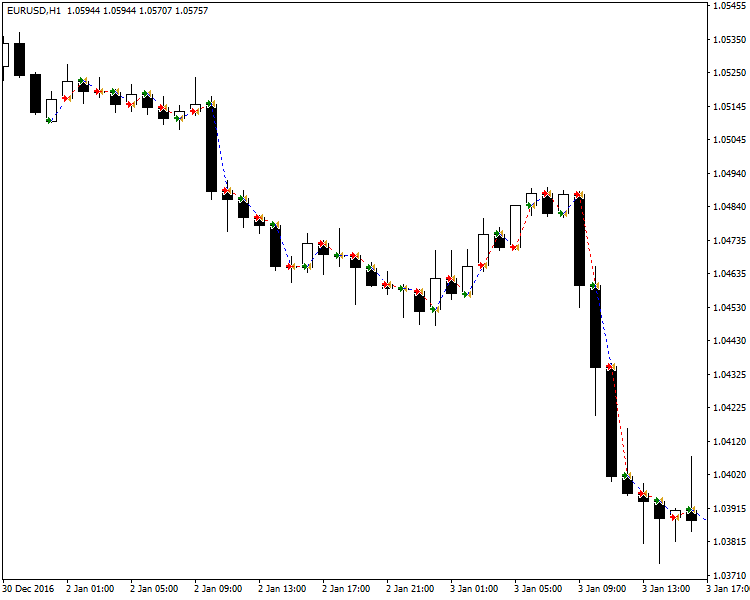(MT5)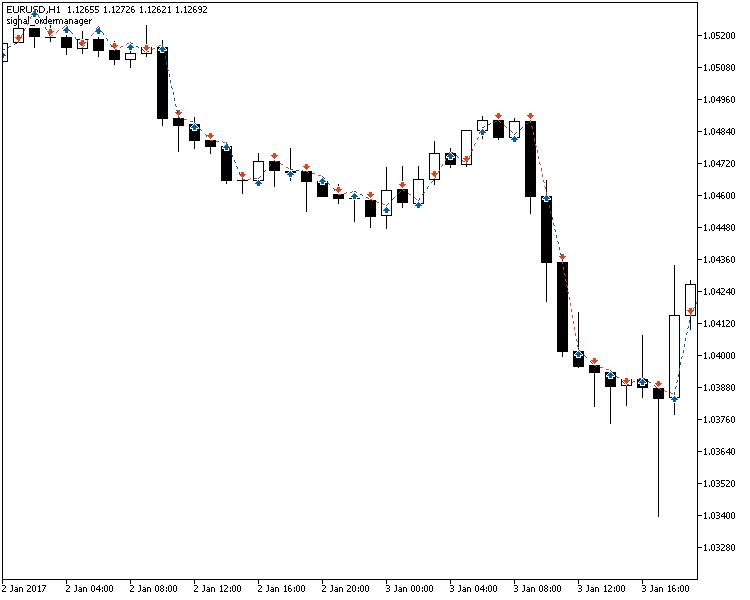#### Example #2: MA Expert Advisor

Our next example involves the use of the Moving Average indicator in the evaluation of trading signals. MA is a standard indicator in both MetaTrader 4 and MetaTrader 5, and so is one of the most simple indicators we can use when coding a cross-platform expert advisor.

Similar to the previous example, we create a custom signal by extending CSignal:

```class SignalMA: public CSignal
{
protected:
CiMA             *m_ma;
CSymbolInfo      *m_symbol;
string            m_symbol_name;
ENUM_TIMEFRAMES   m_timeframe;
int               m_signal_bar;
double            m_close;
//rest of the class```

As we can see, both MQL4 and MQL5 libraries already provided a class object for the Moving Average indicator. This will make it easier to incorporate the indicator to our custom signal class. Although it may not be necessary, in this example, we will also store the target symbol through m_symbol, a pointer to a CSybmolInfo object. We also declare a variable named m_close, where the value of the close price of the signal bar will be stored. The rest of the protected class members are the parameters for the moving average indicator.

The previous example does not have a complex data structure to prepare before being used. In this example, however, there is (the indicator), and so we would have to initialize it on the class constructor:

```void SignalMA::SignalMA(const string symbol,const ENUM_TIMEFRAMES timeframe,const int period,const int shift,const ENUM_MA_METHOD method,const ENUM_APPLIED_PRICE applied,const int bar)
{
m_symbol_name= symbol;
m_timeframe = timeframe;
m_signal_bar = bar;
m_ma=new CiMA();
m_ma.Create(symbol,timeframe,period,0,method,applied);
}```

Getting the signal of the moving average often involves the comparison of its value to a certain price on the chart. It can be a specific price on the chart, like the open or close, or the current bid and ask prices. The latter will require a symbol object to work with. In this example, we will extend the Init method as well so as to initialize get the correct symbol to use from the symbol manager, for those who would like to use bid and ask prices for comparison rather than OHLC data (and their derivatives).

```bool SignalMA::Init(CSymbolManager *symbol_man,CEventAggregator *event_man=NULL)
{
if(CSignal::Init(symbol_man,event_man))
{
if(CheckPointer(m_symbol_man))
{
m_symbol=m_symbol_man.Get();
if(CheckPointer(m_symbol))
return true;
}
}
return false;
}```

The next method to extend is the calculate method, as shown in the following code:

```bool SignalMA::Calculate(void)
{
double close[];
if(CopyClose(m_symbol_name,m_timeframe,signal_bar,1,close)>0)
{
m_close=close;
return true;
}
return false;
}```

There is no longer any need to refresh the data of the indicators, as this is already performed within Refresh method of CSignal. Alternatively, we may also implement the CSignal class descendant so that to get the close price of the signal bar, we use the class CCloseBuffer. It is also of a descendant of CSeries, so we can add it to m_indicators so that the CCloseBuffer instance would also be refreshed along with the other indicators. In that case, there is no longer any need to extend either the Refresh or Calculate methods of CSignal.

For this particular signal, there is no need to further extend the Update method, so let us proceed to the actual generation of the signal itself. The following code snippets show the methods for LongCondition and ShortCondition:

```bool SignalMA::LongCondition(void)
{
return m_close>m_ma.Main(m_signal_bar);
}

bool SignalMA::ShortCondition(void)
{
return m_close<m_ma.Main(m_signal_bar);
}```

The conditions are very simple: if the close price of the signal bar is greater than the moving average value at the signal bar, it's a long signal. On the other hand, if the close price is lesser, then we have a short signal.

Similar to the previous example, we simply initialize all the other needed pointers and then add the CSignal instance to its container (CSignals instance). The following shows the additional code needed for the initialization of the signal under OnInit:

```SignalMA *signal_ma=new SignalMA(Symbol(),(ENUM_TIMEFRAMES) Period(),maperiod,0,mamethod,maapplied,signal_bar);
signals=new CSignals();
signals.Init(GetPointer(symbol_manager),NULL);```

The following code shows the OnTick function, which is the same as the OnTick function found on the previous example:

```void OnTick()
{
//---
symbol_info.RefreshRates();
signals.Check();
if(signals.CheckOpenLong())
{
close_last();
}
else if(signals.CheckOpenShort())
{
close_last();
}
}```

The following screen shots are the results of a test of the expert advisor on MT4 and MT5. As we can see, the expert advisors execute the same logic:

(MT4)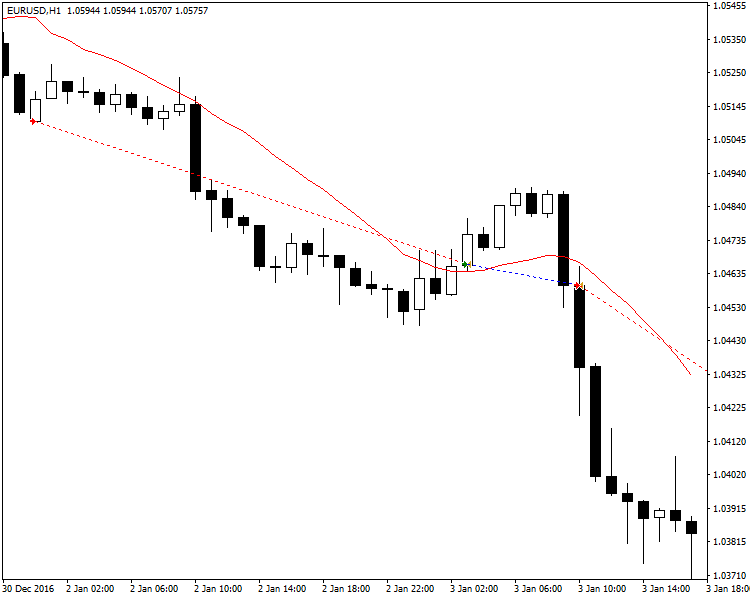(MT5)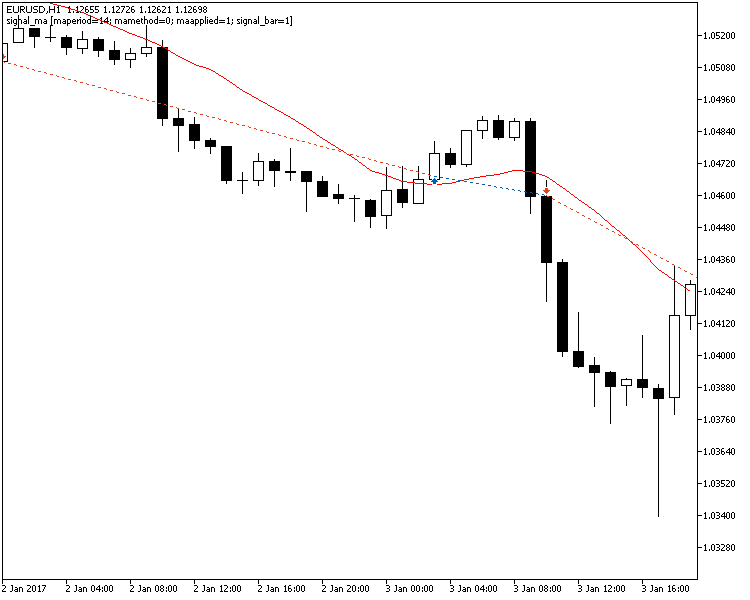#### Example #3: HA Expert Advisor

In our next example, we will deal with the use of the Heiken Ashi indicator in an EA. Unlike the Moving Average indicator, the Heiken Ashi indicator is a custom indicator, so coding this expert advisor will be slightly more complicated than the previous example, since we also need to declare class for the Heiken Ashi indicator by extending CiCustom. To begin, let us show the class definition for CiHA, our class object for the HA indicator:

```class CiHA: public CiCustom
{
public:
CiHA(void);
~CiHA(void);
bool              Create(const string symbol,const ENUM_TIMEFRAMES period,
const ENUM_INDICATOR type,const int num_params,const MqlParam &params[],const int buffers);
double            GetData(const int buffer_num,const int index) const;
};```

There are two methods that we need to extend, the Create and GetData methods. For the Create method, we need to redefine the class constructor since other tasks are needed to be performed to prepare the instance of the indicator (initialization, as can be seen when standard indicator objects extend from CIndicator):

```bool CiHA::Create(const string symbol,const ENUM_TIMEFRAMES period,const ENUM_INDICATOR type,const int num_params,const MqlParam &params[],const int buffers)
{
NumBuffers(buffers);
if(CIndicator::Create(symbol,period,type,num_params,params))
return Initialize(symbol,period,num_params,params);
return false;
}```

Here, we declare the number of buffers the indicator is supposed to have, and then Initialize the parameters passed to it. The parameters of the indicator is stored in a structure (MqlParam).

For the GetData method, the two languages differ in implementation. In MQL4, a direct call is made to the iCustom function which gives the value of the indicator at a particular bar on the chart. On the other hand, in MQL5, the process of calling indicator is different. iCustom gives a handle to the indicator (roughly similar to what is done in file operations). To access the value of the MetaTrader 5 indicator at a certain bar, one should use that handle, and not a call to the iCustom function. In this case, we split the implementation:

```double CiHA::GetData(const int buffer_num,const int index) const
{
#ifdef __MQL5__
return CiCustom::GetData(buffer_num,index);
#else
return iCustom(m_symbol,m_period,m_params.string_value,buffer_num,index);
#endif
}```

Note that in this method, for the MQL5 version, we are simply returning the result of a call to the parent method (CiCustom). On the other hand, in MQL4, the parent method (CiCustom) only returns zero, and so we have to extend it by actually making the call to the MQL4 iCustom function. Since this MQL4 function does not use a structure (MqlParams) to store the indicator parameters, the call to this functions would almost always be different for each custom indicator.

In the extension of CSignal for this EA, there is not much difference in comparison with the previous examples. For the constructor, we simply redefine the arguments to the method to accommodate for the parameters of the indicator(s) needed for the evaluation of the signal. For this particular signal, we only use one indicator:

```void SignalHA::SignalHA(const string symbol,const ENUM_TIMEFRAMES timeframe,const int numparams,const MqlParam &params[],const int bar)
{
m_symbol_name= symbol;
m_signal_bar = bar;
m_ha=new CiHA();
m_ha.Create(symbol,timeframe,IND_CUSTOM,numparams,params,4);
}```

For the Calculate method, we also need to split the implementation, since the Heiken Ashi indicators for MetaTrader 4 and MetaTrader 5 differ in the arrangement of their buffers. For the former, it's Low/High, High/Low, Open, and Close that occupy the first (buffer 0), second, third, and fourth buffers. On the other hand, for the MQL5 version, the arrangement is Open, High, Low, and Close. Thus, we have to consider the particular buffer to access when getting a value from the indicator, depending on the platform being used:

```bool SignalHA::Calculate(void)
{
#ifdef __MQL5__
m_open=m_ha.GetData(0,signal_bar);
#else
m_open=m_ha.GetData(2,signal_bar);
#endif
m_close=m_ha.GetData(3,signal_bar);
return true;
}```

For the MQL5 version, the open price of the HA candle is from the first buffer (buffer 0), while for the MQL4 version, it can be found on the 3rd buffer(buffer 2). The close price of the HA candle can be found on the fourth buffer (buffer 3) for both versions, so we put the statement outside of the preprocessor declaration.

For the evaluation of signals, we always have to update the LongCondition and ShortCondition methods depending on what particular criteria will be used to evaluate the stored values. For this we use the typical use of Heiken Ashi by checking if the signal bar is bullish or bearish:

```bool SignalHA::LongCondition(void)
{
return m_open<m_close;
}

bool SignalHA::ShortCondition(void)
{
return m_open>m_close;
}```

The OnTick function for this expert advisor will be similar to those from previous examples, so let us move on with the OnInit function:

```int OnInit()
{
//---
order_manager=new COrderManager();
symbol_manager=new CSymbolManager();
symbol_info=new CSymbolInfo();
if(!symbol_info.Name(Symbol()))
Print("symbol not set");
order_manager.Init(symbol_manager,NULL);

MqlParam params;
params.type=TYPE_STRING;
#ifdef __MQL5__
params.string_value="Examples\\Heiken_Ashi";
#else
params.string_value="Heiken Ashi";
#endif
SignalHA *signal_ha=new SignalHA(Symbol(),0,1,params,signal_bar);
signals=new CSignals();
signals.Init(GetPointer(symbol_manager),NULL);
//---
return(INIT_SUCCEEDED);
}```

Here we notice that the location of the Heiken Ashi indicator ex4 file is different depending on the trading platform used. Since MqlParams requires that the first parameter to store should be the name of the custom indicator (without the extension), once again, we will need to split the implementation in specifying the first parameter. In MQL5, the indicator can be found by default under "Indicators\Examples\Heiken Ashi" while in MQL4, the indicator can be found under "Indicators\Heiken Ashi".

The following screen shots shows the results of a test of the expert advisor on MetaTrader 4 and MetaTrader 5. As shown, although the indicator somehow differ in the way they plot on the chart, we can see that both indicators have the same logic, and that the expert advisor for both versions were able to execute based on the same logic:

(MT4)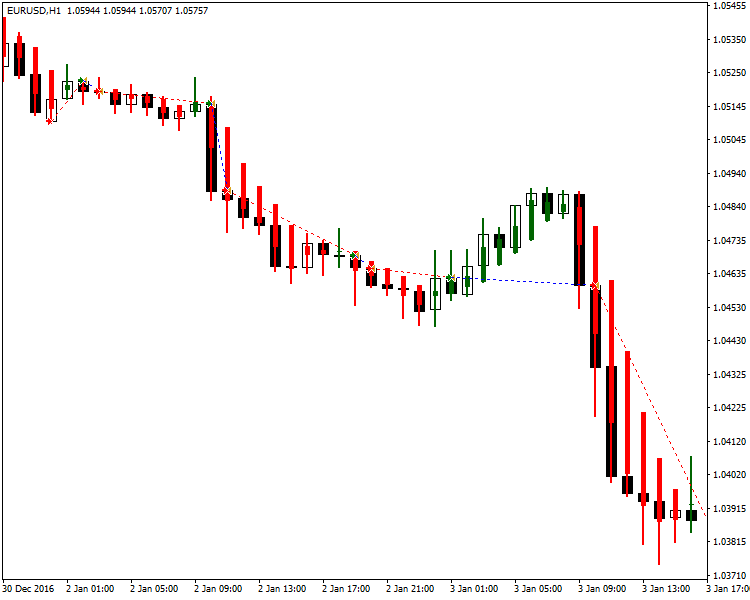(MT5)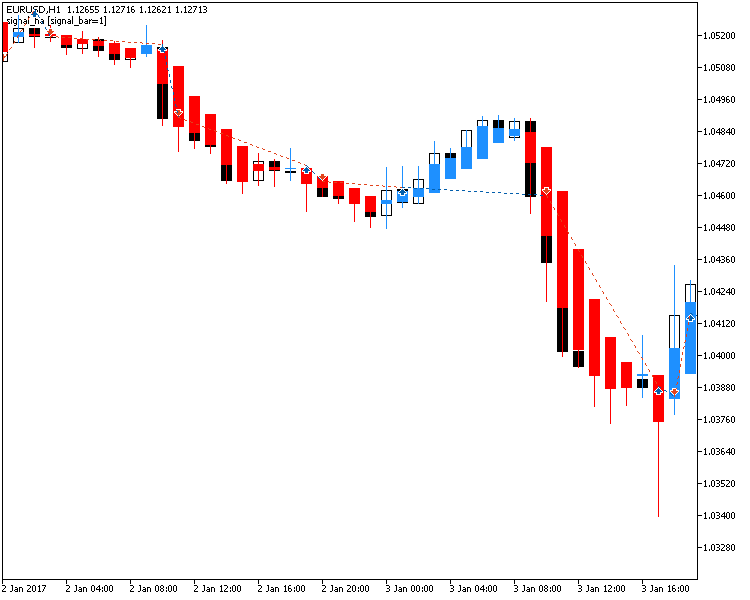#### Example #4: An Expert Advisor Based on HA and MA

Our last example is the combination of the MA and HA indicators to be included in the EA. There is not much difference with this example. We simply add the class definitions found in the 2nd and 3rd examples, and then add the pointers to instances of CSignalMA and CSignalHA to an instance of CSignals. The following shows a result of a test using this expert advisor.

(MT4)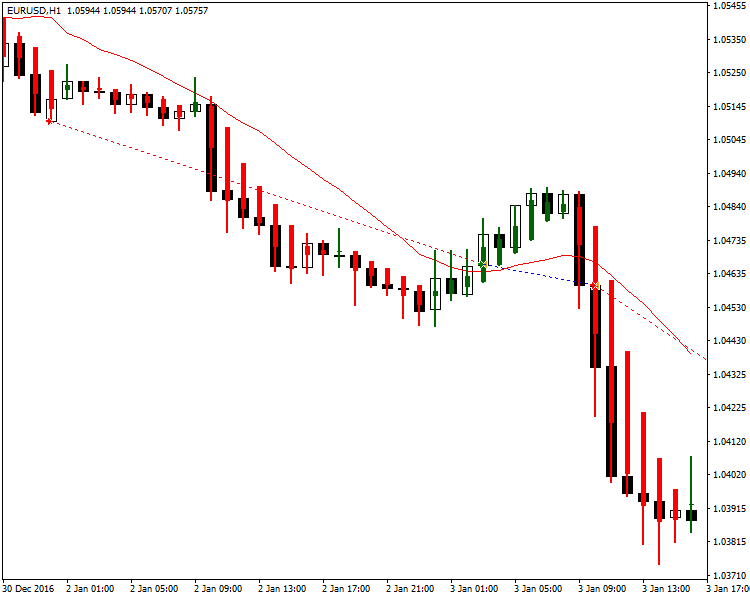(MT5)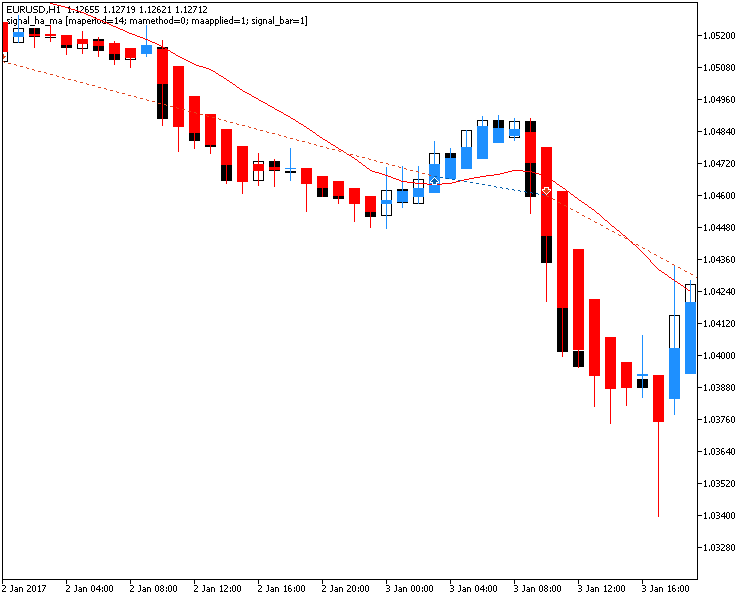### Conclusion

In this article, we have discussed the CSignal and CSignals classes, which are the class objects to be used for handling the evaluation of the overall signal of a cross-platform expert advisor in a given tick. The said classes were designed to the processes involved in the evaluation of signals are segregated from the rest of the code of the expert advisor.

Attached files |
signals.zip (3907.49 KB)
Last comments | Go to discussion (28)| 2 Apr 2018 at 16:40

Also, regarding the OrdersTotal() in MT5, it only counts the pending orders (not the equivalent of MT4's OrdersTotal). The code in TradeOpen for OrderManager:

```bool COrderManager::TradeOpen(const string symbol,ENUM_ORDER_TYPE type,double price,bool in_points=true)
{
bool ret=false;
double lotsize=0.0;
int orders_total = OrdersTotal();```

uses the OrdersTotal() method of the class, which is just a wrapper of COrders' method (also the same name). COrders extends CArrayObj, so it is not really using the MT5 native OrdersTotal() function. The above is also equivalent to using:

`int orders_total = this.OrdersTotal();`

On the other hand, if we use:

`int orders_total = ::OrdersTotal();`

this explicitly calls the MT5 native function. You may already be familiar with this, but just in case for other people who encounter a similar problem.| 2 Apr 2018 at 20:43

Thank you Enrico. The issue disappeared.

/Karl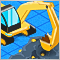| 3 Apr 2018 at 06:26
Enrico Lambino:
```#ifdef __MQL5__
if (!(MQLInfoInteger(MQL_TESTER) || MQLInfoInteger(MQL_OPTIMIZATION))) // MQL4-code, not only MQL5.
#else
if (!(IsTesting() || IsOptimization()))
#endif```
`MQLInfoInteger(MQL_TESTER) || MQLInfoInteger(MQL_OPTIMIZATION) == MQLInfoInteger(MQL_TESTER)`| 3 Apr 2018 at 11:20
fxsaber:
I see. Yes, you are right.| 7 Aug 2019 at 09:24

Hi Enrico,

In the Github at iceron/MQLx, there is a change notice of m_new_signal and m_new_signal_close.

m_signal_new can be changed by the void CSignalsBase::NewSignal(const bool value) method, but there are no method available to change m_new_signal_close.

Could you please elaborate on the usage of m_new_signal and m_new_signal_close?

```   if(m_invert)
{
CSignal::SignalInvert(m_signal_open);
CSignal::SignalInvert(m_signal_close);
}
if(m_new_signal)
{
if(m_signal_open==m_signal_open_last)
m_signal_open = CMD_NEUTRAL;
}
if(m_new_signal_close)
{
if(m_signal_close==m_signal_close_last)
m_signal_close = CMD_NEUTRAL;
}```

Best Regards/

Karl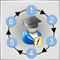MQL5 Cookbook - Creating a ring buffer for fast calculation of indicators in a sliding window

The ring buffer is the simplest and the most efficient way to arrange data when performing calculations in a sliding window. The article describes the algorithm and shows how it simplifies calculations in a sliding window and makes them more efficient.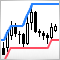Trading with Donchian Channels

In this article, we develop and tests several strategies based on the Donchian channel using various indicator filters. We also perform a comparative analysis of their operation.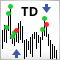Thomas DeMark's Sequential (TD SEQUENTIAL) using artificial intelligence

In this article, I will tell you how to successfully trade by merging a very well-known strategy and a neural network. It will be about the Thomas DeMark's Sequential strategy with the use of an artificial intelligence system. Only the first part of the strategy will be applied, using the Setup and Intersection signals.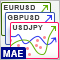Analyzing Balance/Equity graphs by symbols and EAs' ORDER_MAGIC

With the introduction of hedging, MetaTrader 5 provides an excellent opportunity to trade several Expert Advisors on a single trading account simultaneously. When one strategy is profitable, while the second one is loss-making, the profit graph may hang around zero. In this case, it is useful to build the Balance and Equity graphs for each trading strategy separately.